Prime Factorization 5
Set 5 (10 Questions)
Determine the prime factors of all numbers through 50 and write the numbers as the product of their prime factors by using exponents to show multiples of a factor.
From Mr. Anker Tests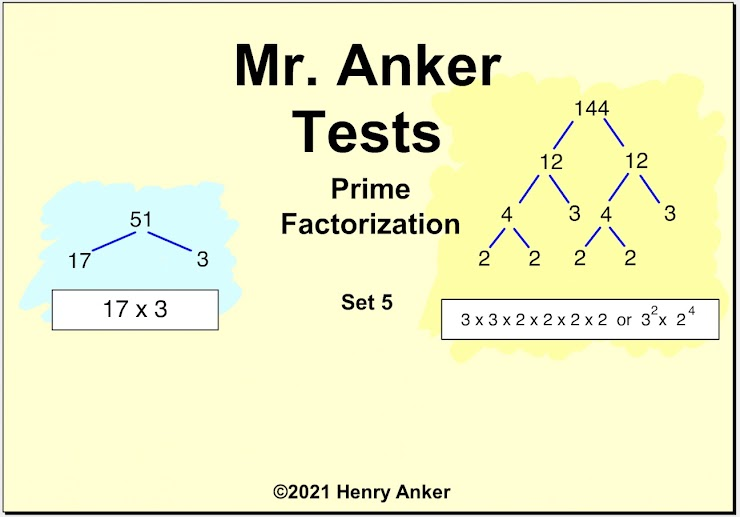Prime Factorization Help Slide 1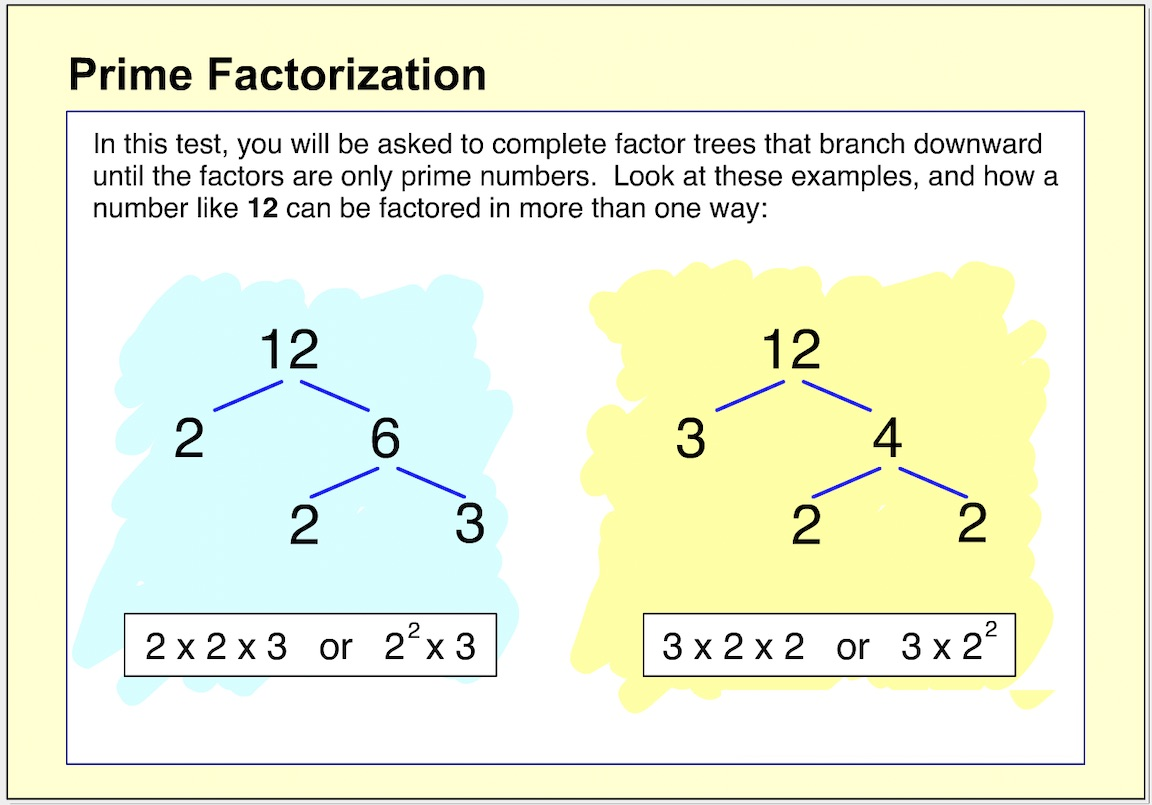Prime Factorization Help Slide 2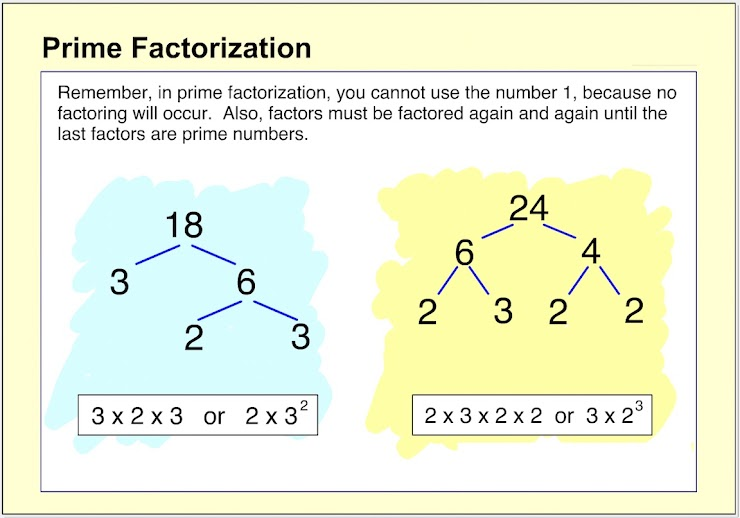Prime Factorization Help Slide 3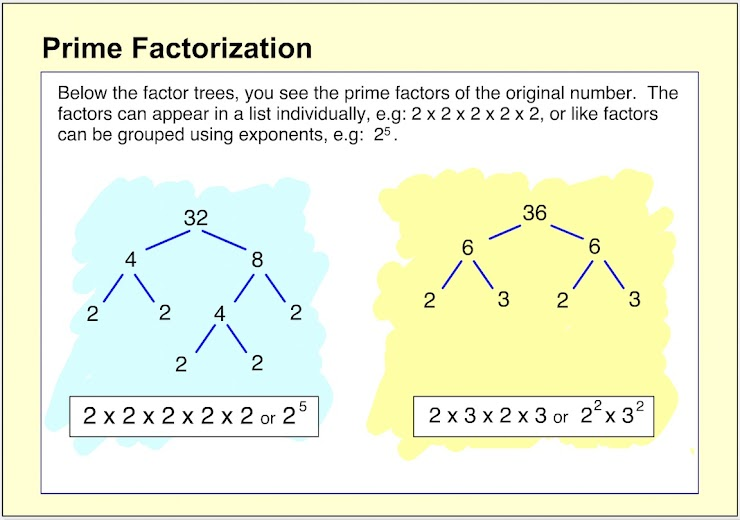Prime Factorization Help Slide 4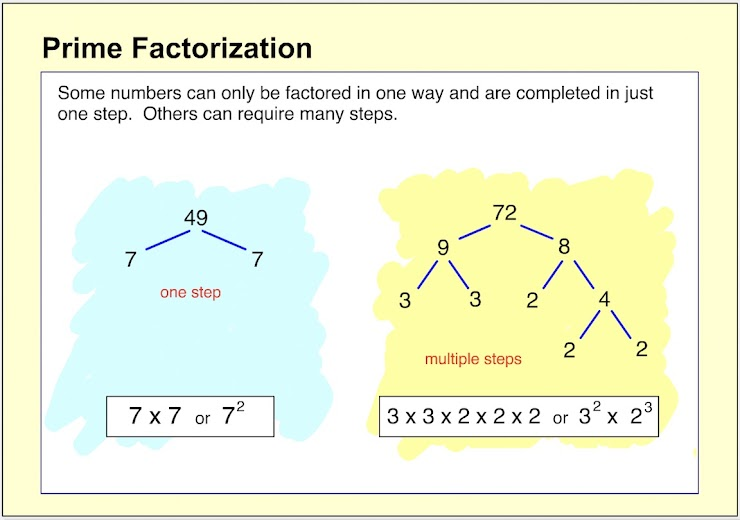Prime Factorization Help Slide 5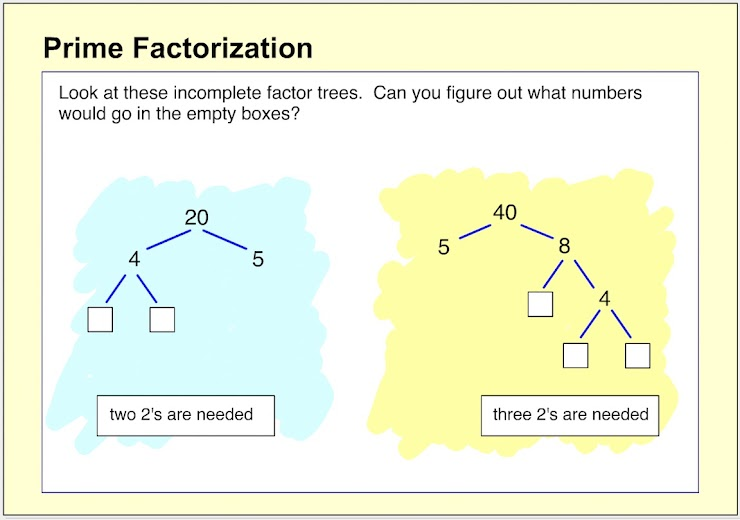1.  On the line below, type in the exponent value to complete the prime factorization. *
1 point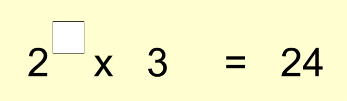2. On the line below, type in the exponent value to complete the prime factorization. *
1 point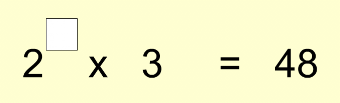3. On the line below, type in the exponent value to complete the prime factorization. *
1 point4. On the line below, type in the whole number needed to complete the prime factorization. *
1 point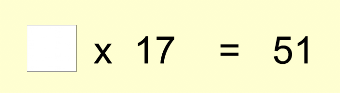5.  What is the prime factorization of 80? *
1 point
6.  What is the prime factorization of 144? *
1 point
7.  What is the prime factorization of 200? *
1 point
8.  What is the prime factorization of 39? *
1 point
9.  Which of the following is -not- a prime number? *
1 point
10.  What is the prime factorization of 81? *
1 point
Submit
Clear form